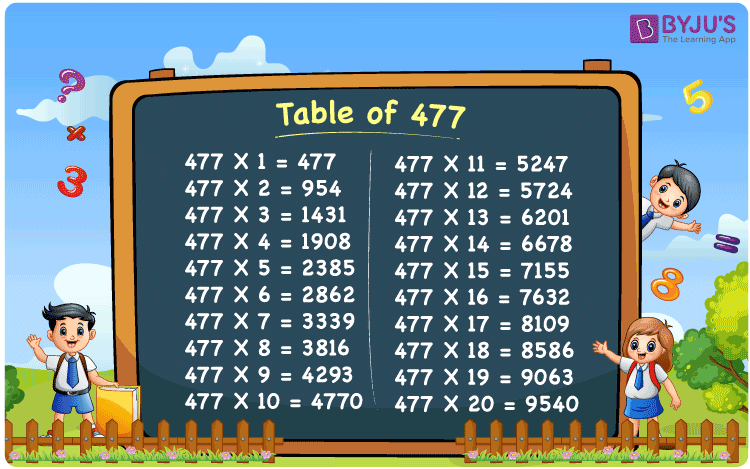Checkout JEE MAINS 2022 Question Paper Analysis : Checkout JEE MAINS 2022 Question Paper Analysis :

# Table of 477

The Table of 477 is provided in a tabular format to help students quickly check the values of multiples of 477. The multiplication table of 477 up to 20 is available in a PDF format to download, which will be handy while solving problems related to the 477 times table. The table of 477 will be useful while doing mental maths, which is a necessary skill for appearing in any competitive examination.

## Table of 477 Chart

The 477 times table chart up to 20 is given here with an attractive image that can be used as a flashcard to learn the times table of 477. The multiplication table of 477 can be useful for many complex arithmetic calculations.## What is the 477 Times Table?

The 477 times table is the multiplication of 477 with any natural number. The 477 times table can be obtained by multiplication and also by adding 477 repeatedly. The below chart shows the multiplication table of 477, in both multiplication and addition form.

 477×1 = 477 477 477×2 = 954 477 + 477 = 954 477×3 = 1431 477 + 477 + 477 = 1431 477×4 = 1908 477 + 477 + 477 + 477 = 1908 477×5 = 2385 477 + 477 + 477 + 477 + 477 = 2385 477×6 = 2862 477 + 477 + 477 + 477 + 477 + 477 = 2862 477×7 = 3339 477 + 477 + 477 + 477 + 477 + 477 + 477 = 3339 477×8 = 3816 477 + 477 + 477 + 477 + 477 + 477 + 477 + 477 = 3816 477×9 = 4293 477 + 477 + 477 + 477 + 477 + 477 + 477 + 477 + 477 = 4293 477×10 = 4770 477 + 477 + 477 + 477 + 477 + 477 + 477 + 477 + 477 + 477 = 4770

## Multiplication Table of 477

Given below are the first 20 multiples of 477 that are obtained by the multiplication of the first 20 natural numbers with 477.

 477 × 1 = 477 477 × 2 = 954 477 × 3 = 1431 477 × 4 = 1908 477 × 5 = 2385 477 × 6 = 2862 477 × 7 = 3339 477 × 8 = 3816 477 × 9 = 4293 477 × 10 = 4770 477 × 11 = 5247 477 × 12 = 5724 477 × 13 = 6201 477 × 14 = 6678 477 × 15 = 7155 477 × 16 = 7632 477 × 17 = 8109 477 × 18 = 8586 477 × 19 = 9063 477 × 20 = 9540

## Solved Examples on the Table of 477

Example 1:

What is 4 more than the fourth multiple of 477?

Solution:

The fourth multiple of 477 = 477 × 4 = 1908

Four more than 1908 = 1908 + 4 = 1912.

## Frequently Asked Questions on the Table of 477

### What is the times tables of 477?

The 477 times table is the multiplication of 477 with natural numbers. Starting with 477 × 1 = 477, 477 × 2 = 954, 477 × 3 = 1431, 477 × 4 = 1908, 477 × 5 = 2385 and so on.

### What times tables has 477?

477 comes in the times tables of 1, 3, 9, 53, 159, 477 and 477.

### How many times 3 is 477?

159 times 3, 159 × 3 = 477.